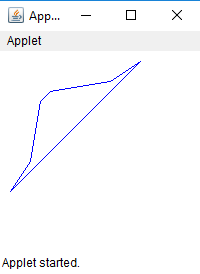# Draw a Polygon in Java Applet

Polygon is a closed figure with finite set of line segments joining one vertex to the other. The polygon comprises of set of (x, y) coordinate pairs where each pair is the vertex of the polygon. The side of the polygon is the line drawn between two successive coordinate pairs and a line segment is drawn from the first pair to the last pair.

We can draw Polygon in java applet by three ways :

1. drawPolygon(int[] x, int[] y, int numberofpoints) : draws a polygon with the given set of x and y points.

 `// Java program to draw polygon using ` `// drawPolygon(int[] x, int[] y, int numberofpoints) ` `// function ` `import` `java.awt.*; ` `import` `javax.swing.*; ` ` `  `public` `class` `poly ``extends` `JApplet { ` ` `  `    ``// called when applet is started ` `    ``public` `void` `init() ` `    ``{ ` `        ``// set the size of applet to 300, 300 ` `        ``setSize(``200``, ``200``); ` `        ``show(); ` `    ``} ` ` `  `    ``// invoked when applet is started ` `    ``public` `void` `start() ` `    ``{ ` `    ``} ` ` `  `    ``// invoked when applet is closed ` `    ``public` `void` `stop() ` `    ``{ ` `    ``} ` ` `  `    ``public` `void` `paint(Graphics g) ` `    ``{ ` `        ``// x coordinates of vertices ` `        ``int` `x[] = { ``10``, ``30``, ``40``, ``50``, ``110``, ``140` `}; ` ` `  `        ``// y coordinates of vertices ` `        ``int` `y[] = { ``140``, ``110``, ``50``, ``40``, ``30``, ``10` `}; ` ` `  `        ``// number of vertices ` `        ``int` `numberofpoints = ``6``; ` ` `  `        ``// set the color of line drawn to blue ` `        ``g.setColor(Color.blue); ` ` `  `        ``// draw the polygon using drawPolygon function ` `        ``g.drawPolygon(x, y, numberofpoints); ` `    ``} ` `} `

Output :2. drawPolygon(Polygon p) : draws a polygon with the given object of Polygon class.

 `// Java program to draw polygon ` `// using drawPolygon(Polygon p) ` `// function ` `import` `java.awt.*; ` `import` `javax.swing.*; ` ` `  `public` `class` `poly ``extends` `JApplet { ` ` `  `    ``// called when applet is started ` `    ``public` `void` `init() ` `    ``{ ` `        ``// set the size of applet to 300, 300 ` `        ``setSize(``200``, ``200``); ` `        ``show(); ` `    ``} ` ` `  `    ``// invoked when applet is started ` `    ``public` `void` `start() ` `    ``{ ` `    ``} ` ` `  `    ``// invoked when applet is closed ` `    ``public` `void` `stop() ` `    ``{ ` `    ``} ` ` `  `    ``public` `void` `paint(Graphics g) ` `    ``{ ` `        ``// x coordinates of vertices ` `        ``int` `x[] = { ``10``, ``30``, ``40``, ``50``, ``110``, ``140` `}; ` ` `  `        ``// y coordinates of vertices ` `        ``int` `y[] = { ``140``, ``110``, ``50``, ``40``, ``30``, ``10` `}; ` ` `  `        ``// number of vertices ` `        ``int` `numberofpoints = ``6``; ` ` `  `        ``// create a polygon with given x, y coordinates ` `        ``Polygon p = ``new` `Polygon(x, y, numberofpoints); ` ` `  `        ``// set the color of line drawn to blue ` `        ``g.setColor(Color.blue); ` ` `  `        ``// draw the polygon using drawPolygon ` `        ``// function using object of polygon class ` `        ``g.drawPolygon(p); ` `    ``} ` `} `

Output :3. drawLine(int x, int y, int x1, int y1) : In this method we would connect adjacent vertices with a line segment and also connect the first and last vertex.

 `// Java code to draw a polygon ` `// using  drawLine(int x, int y, int x1, int y1) ` `// function ` `import` `java.awt.*; ` `import` `javax.swing.*; ` ` `  `public` `class` `poly ``extends` `JApplet { ` ` `  `    ``// called when applet is started ` `    ``public` `void` `init() ` `    ``{ ` `        ``// set the size of applet to 300, 300 ` `        ``setSize(``200``, ``200``); ` `        ``show(); ` `    ``} ` ` `  `    ``// invoked when applet is started ` `    ``public` `void` `start() ` `    ``{ ` `    ``} ` ` `  `    ``// invoked when applet is closed ` `    ``public` `void` `stop() ` `    ``{ ` `    ``} ` ` `  `    ``public` `void` `paint(Graphics g) ` `    ``{ ` `        ``// x coordinates of vertices ` `        ``int` `x[] = { ``10``, ``30``, ``40``, ``50``, ``110``, ``140` `}; ` ` `  `        ``// y coordinates of vertices ` `        ``int` `y[] = { ``140``, ``110``, ``50``, ``40``, ``30``, ``10` `}; ` ` `  `        ``// number of vertices ` `        ``int` `numberofpoints = ``6``; ` ` `  `        ``// set the color of line drawn to blue ` `        ``g.setColor(Color.blue); ` ` `  `        ``// join the adjacent vertices ` `        ``for` `(``int` `i = ``0``; i < numberofpoints - ``1``; i++) ` `            ``g.drawLine(x[i], y[i], x[i + ``1``], y[i + ``1``]); ` ` `  `        ``// join the first and last vertex ` `        ``g.drawLine(x[``0``], y[``0``], x[numberofpoints - ``1``], y[numberofpoints - ``1``]); ` `    ``} ` `} `

Output :Note: The above function are a part of java.awt package and belongs to java.awt.Graphics class. Also, these codes might not run in an online compiler please use an offline compiler. The x and y coordinates can be changed by the programmer according to their needMy Personal Notes arrow_drop_upCheck out this Author's contributed articles.

If you like GeeksforGeeks and would like to contribute, you can also write an article using contribute.geeksforgeeks.org or mail your article to contribute@geeksforgeeks.org. See your article appearing on the GeeksforGeeks main page and help other Geeks.

Please Improve this article if you find anything incorrect by clicking on the "Improve Article" button below.

Article Tags :
Practice Tags :

2

Please write to us at contribute@geeksforgeeks.org to report any issue with the above content.